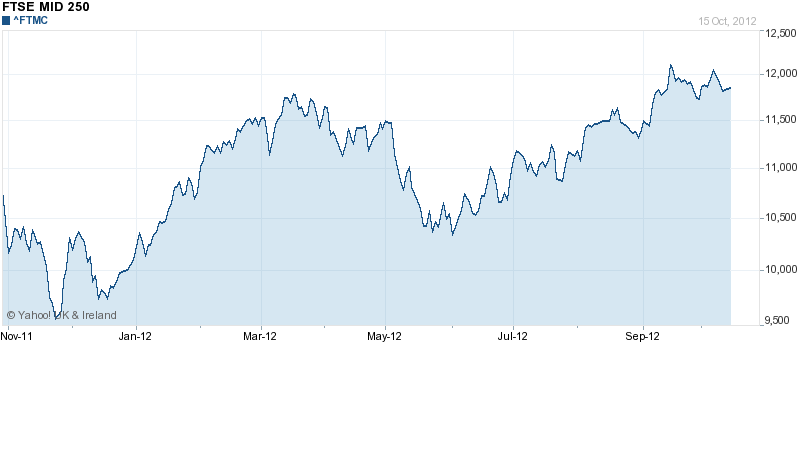Want to share your content on R-bloggers? click here if you have a blog, or here if you don't.

The Brownian motion is certainly the most famous stochastic process (a random variable evolving in the time). It has been the first way to model a stock option price (Louis Bachelier’s thesis in 1900).

The reason why is easy to understand, a Brownian motion is graphically very similar to the historical price of a stock option.The historical price of the index FTSE MID 250, source Yahoo Finance.

Even though it is not any more considered as the real distribution of stock options, it is still used in finance and methods to simulate a Brownian motion are really useful. As you will see the code is strikingly easy. We have used the Donsker’s principle.

If Y(i) is a serie of k variables normally distributed, then we can  create X(i) = (Y(1) + … + Y(i))sqrt(i/k). Then X(i) is a proxy of the Brownian motion.

The code (R) :

size = 1000
y = rnorm(size)
x = y

for (i in 1:size){
x[i] = 1/sqrt(size)*(sum(y[1:i])*sqrt(i))
}

plot(x, type = ‘l’, xlab = ‘Time’, ylab = ‘Profit’)